Courses

# Fourier Series and Transform Civil Engineering (CE) Notes | EduRev

## Civil Engineering (CE) : Fourier Series and Transform Civil Engineering (CE) Notes | EduRev

The document Fourier Series and Transform Civil Engineering (CE) Notes | EduRev is a part of the Civil Engineering (CE) Course Topic wise GATE Past Year Papers for Civil Engineering.
All you need of Civil Engineering (CE) at this link: Civil Engineering (CE)

Question 1: The solution at x = 1, t = 1 of the partial differential equation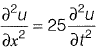subject to initial conditions of u(0)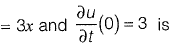______.
(a) 1
(b) 2
(c) 4
(d) 6

[2018 : 2 Marks, Set-I]
Answer: (d)
Solution: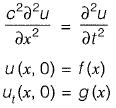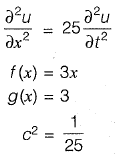D’Alembert’s formula,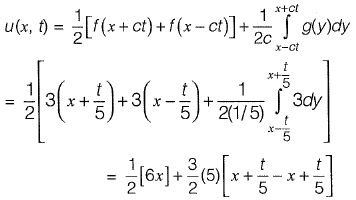u(x, t) = 3x + 3t
At x = 1, t = 1
u(x, f) = 6

Question 2: The Fourier series of the function,

f(x) = 0, (-π < x < 0 )

f(x) = π - x, (0 < x < π)   in the interval [- π, π] is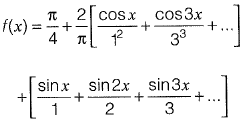The convergence of the above Fourier series at x = 0 gives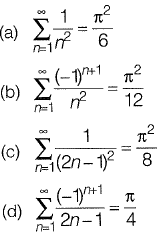[2016 : 1 Mark, Set-II]
Answer: (c)
Solution: The function is f(x) = 0,
-p < x < 0
= p - x, 0 < x < π
And Fourier series is,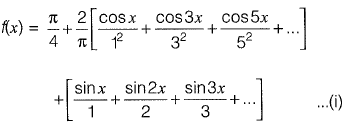At x = 0, (a point of discontinuity), the fourier series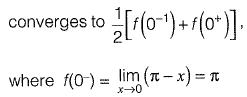Hence, eq. (i), we get,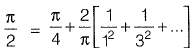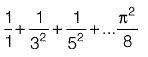Question 3: The infinite series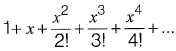corresponds to
(a) secx
(b) ex
(c) cos x
(d) 1 + sin2x
[2011 : 2 Marks]

Answer: (b)
Solution: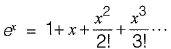(By McLaurin’s series expansion)

Offer running on EduRev: Apply code STAYHOME200 to get INR 200 off on our premium plan EduRev Infinity!

69 docs

,

,

,

,

,

,

,

,

,

,

,

,

,

,

,

,

,

,

,

,

,

;